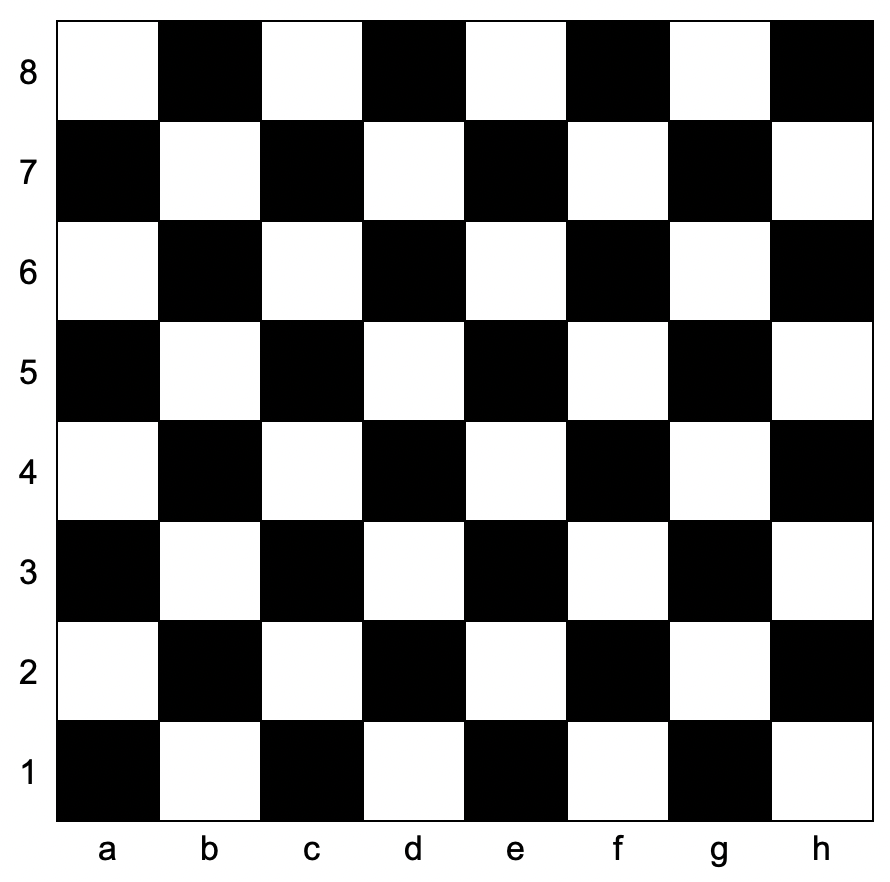# 判断国际象棋棋盘中一个格子的颜色

## 1812. 判断国际象棋棋盘中一个格子的颜色 (Easy)```输入：coordinates = "a1"

```

```输入：coordinates = "h3"

```

```输入：coordinates = "c7"

```

• `coordinates.length == 2`
• `'a' <= coordinates <= 'h'`
• `'1' <= coordinates <= '8'`

[字符串]